# DAV Class 3 Maths Chapter 11 Worksheet 1 Solutions

The DAV Maths Book Class 3 Solutions and DAV Class 3 Maths Chapter 11 Worksheet 1 Solutions of Fractions offer comprehensive answers to textbook questions.

## DAV Class 3 Maths Ch 11 WS 1 Solutions

Question 1.
Shade the figures that represent the given fraction.

(a)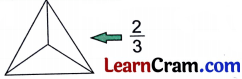Solution: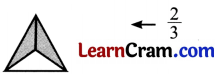(b)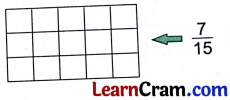Solution:(c)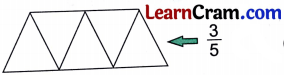Solution:(d)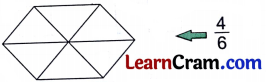Solution: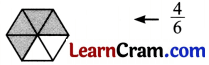(e)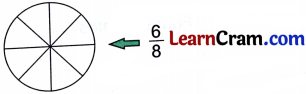Solution: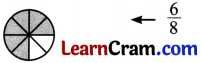Question 2.
Write the fraction to represent the shaded part in each.

(a)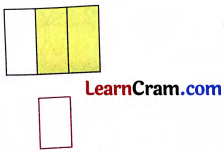Solution:
$$\frac{2}{3}$$

(b)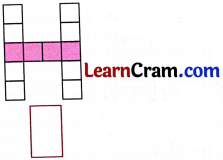Solution:
$$\frac{4}{12}$$

(c)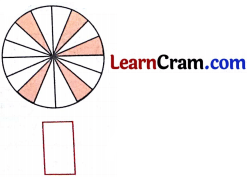Solution:
$$\frac{5}{16}$$Question 3.
Match the following: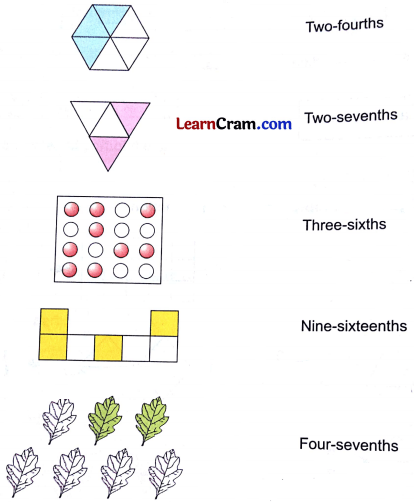Solution: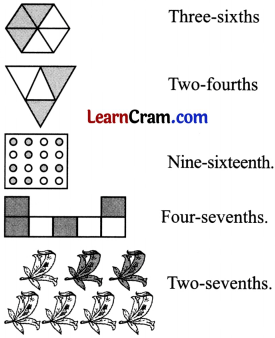Question 4.
From the following collection of figures, indicate the fraction which represents the number of shaded figures in each.

(a)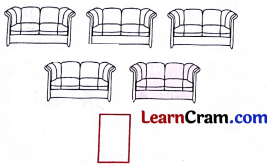Solution:
$$\frac{1}{5}$$

(b)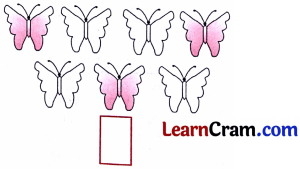Solution:
$$\frac{3}{7}$$

(c)Solution:
$$\frac{3}{6}$$

(d)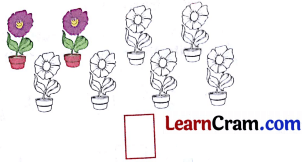Solution:
$$\frac{2}{8}$$Question 5.
Write the fraction in words for each. First one is done for you.

(a) $$\frac{3}{5}$$ = _______
Solution:
Three-fifths

(b) $$\frac{8}{9}$$ = _______
Solution:
Eight-ninths

(c) $$\frac{9}{10}$$ = _______
Solution:
Nine-tenths

(d) $$\frac{5}{7}$$ = _______
Solution:
Five-sevenths

(e) $$\frac{6}{13}$$ = _______
Solution:
Six-thirteenths

(f) $$\frac{10}{19}$$ = _______
Solution:
Ten-Nineteenths.### DAV Class 3 Maths Chapter 11 Worksheet 1 Notes

• When we divide a thing into equal parts then each part is called as a fraction of the whole.
• A fraction can be divided into numerator and denominator.
Numerator (It says how many parts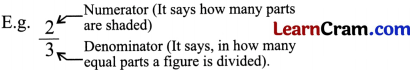• Fractions having same denominators are called like fractions.
• In like fractions we always compare, subtract or add the numerators only. The denominator remains as it is.

Fractions

When a thing is divided into equal parts, each part is called a fraction of the whole.
Suppose a chocolate is divided into two equal parts. Each part is one half of whole chocolate.
It is written as $$\frac{1}{2}$$.
To write a fraction in number = no. of parts chosen / total number of equal parts.In the adjacent fig. We can say $$\frac{1}{2}$$ is shaded. In $$\frac{1}{2}$$ 1 is numerator and 2 is denominator.

One – third:one flag is divided into three equal parts, each part is one – third ($$\frac{1}{3}$$) of whole. Two one – thirds together make two-thirds ($$\frac{2}{3}$$). one-third together make the whole.One-fourth:

When a pizza is divided into four equal-parts, each part is One-fourth ($$\frac{1}{4}$$) of whole.
Similarly,$$\frac{4}{10}$$ of shapes in the above collection are triangle.